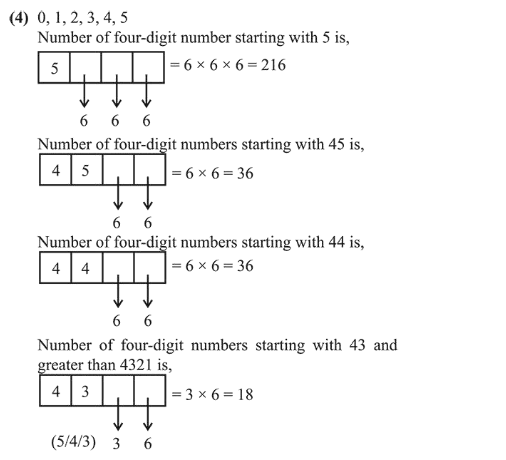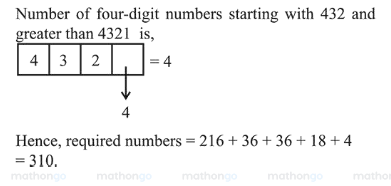# The number of four-digit numbers strictly`
Question:

The number of four-digit numbers strictly greater than 4321 that can be formed using the digits $0,1,2,3,4,5$ (repetition of digits is allowed) is:

1. (1) 288

2. (2) 360

3. (3) 306

4. (4) 310

Correct Option: , 4

Solution: